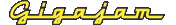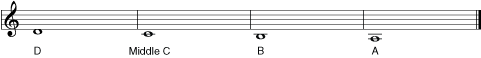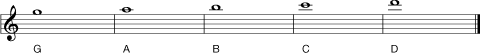EMT Lesson One  How to read music

# Ledger lines

As the notes go further above, or below the stave, ledger lines are added. As the lowest note on the stave is E, the note immediately below the stave is D.

Figure 5. D to A below the stave (treble clef).   (Enlarge)The next note down is C, which has a ledger line through the note head. The next note down from C is a B, where the note head sits just below the ledger line that goes through middle C. The next note down is A, which sits on the second ledger line below the stave. Like middle C, the ledger line goes through the note head of A.

You can see that the notes of A and C sit on the ledger lines below the treble clef and B and D are in the spaces. Ledger lines simply extend the stave when needed. So, it follows that this works in exactly the same way with high notes above the stave.

Figure 6. G to D above the stave in the treble clef   (Enlarge)